# 使用 MQL5 和 MQL4 开发的选择与导航工具: 增加自动模式搜索和显示侦测到的交易品种

21 二月 2019, 08:000
556

### 向自动挑选页面增加功能、

`string panelNamesAddon={"Air Level", "Parabolic", "Gap", "4 weeks Min/Max", "365 days Min/Max", "Round levels", "Mostly Up/Down", "All time high/low", "High=Close"};`

show_panel_buttons 函数就是负责显示选项卡的。它的代码已经修改过了，所有自动挑选选项卡都显示在原有选项卡之后:

```void show_panel_buttons(){
int btn_left=0;
// 定义可以显示选项卡的最大 x 坐标。
int btn_right=(int) ChartGetInteger(0, CHART_WIDTH_IN_PIXELS)-77;
string tmpName="";

for( int i=0; i<ArraySize(panelNames); i++ ){
// 如果新按钮的开始坐标超过了最大可能坐标，
// 转到新的一行
if( btn_left>btn_right-BTN_WIDTH ){
btn_line++;
btn_left=0;
}
// 如果 "homework" 选项卡含有交易品种，就把它们的编号
// 加到选项卡名称中
tmpName=panelNames[i];
if(i>0 && arrPanels[i].Total()>0 ){
tmpName+=" ("+(string) arrPanels[i].Total()+")";
}

// 显示选项卡按钮
ObjectCreate(0, exprefix+"panels"+(string) i, OBJ_BUTTON, 0, 0, 0);
ObjectSetInteger(0,exprefix+"panels"+(string) i,OBJPROP_XDISTANCE,btn_left);
ObjectSetInteger(0,exprefix+"panels"+(string) i,OBJPROP_YDISTANCE,BTN_HEIGHT*btn_line);
ObjectSetInteger(0,exprefix+"panels"+(string) i,OBJPROP_XSIZE,BTN_WIDTH);
ObjectSetInteger(0,exprefix+"panels"+(string) i,OBJPROP_YSIZE,BTN_HEIGHT);
ObjectSetInteger(0,exprefix+"panels"+(string) i,OBJPROP_FONTSIZE,8);
ObjectSetInteger(0,exprefix+"panels"+(string) i,OBJPROP_COLOR,clrBlack);
ObjectSetInteger(0,exprefix+"panels"+(string) i,OBJPROP_BGCOLOR,clrSilver);
ObjectSetString(0,exprefix+"panels"+(string) i,OBJPROP_TEXT,tmpName);
ObjectSetInteger(0,exprefix+"panels"+(string) i,OBJPROP_SELECTABLE,false);
// 如果按钮选项卡当前激活,
// 把它设为按下状态
if( cur_panel == i ){
ObjectSetInteger(0,exprefix+"panels"+(string) i,OBJPROP_STATE, true);
}

btn_left+=BTN_WIDTH;
}
// 如果输入参数中允许显示自动挑选选项卡，就显示它们
for( int i=0; i<ArraySize(panelNamesAddon); i++ ){
if( btn_left>btn_right-BTN_WIDTH ){
btn_line++;
btn_left=0;
}
// 如果选项卡称为 Gap, 就显示当前输入参数的值
// 定义跳空百分比
if(tmpName=="Gap"){
StringAdd(tmpName, " ("+(string) gap_min+"%)");
}

ObjectCreate(0, exprefix+"panels"+(string) (i+ArraySize(panelNames)), OBJ_BUTTON, 0, 0, 0);
ObjectSetInteger(0,exprefix+"panels"+(string) (i+ArraySize(panelNames)),OBJPROP_XDISTANCE,btn_left);
ObjectSetInteger(0,exprefix+"panels"+(string) (i+ArraySize(panelNames)),OBJPROP_YDISTANCE,BTN_HEIGHT*btn_line);
ObjectSetInteger(0,exprefix+"panels"+(string) (i+ArraySize(panelNames)),OBJPROP_XSIZE,BTN_WIDTH);
ObjectSetInteger(0,exprefix+"panels"+(string) (i+ArraySize(panelNames)),OBJPROP_YSIZE,BTN_HEIGHT);
ObjectSetInteger(0,exprefix+"panels"+(string) (i+ArraySize(panelNames)),OBJPROP_FONTSIZE,8);
ObjectSetInteger(0,exprefix+"panels"+(string) (i+ArraySize(panelNames)),OBJPROP_COLOR,clrBlack);
ObjectSetInteger(0,exprefix+"panels"+(string) (i+ArraySize(panelNames)),OBJPROP_BGCOLOR,clrLightSteelBlue);
ObjectSetString(0,exprefix+"panels"+(string) (i+ArraySize(panelNames)),OBJPROP_TEXT,tmpName);
ObjectSetInteger(0,exprefix+"panels"+(string) (i+ArraySize(panelNames)),OBJPROP_SELECTABLE,false);
if( cur_panel == i+ArraySize(panelNames) ){
ObjectSetInteger(0,exprefix+"panels"+(string) (i+ArraySize(panelNames)),OBJPROP_STATE, true);
}

btn_left+=BTN_WIDTH;
}
}

// 如果设置了注释，显示:
if(StringLen(cmt)>0){
string tmpCMT=cmt;
if(from_txt){
StringAdd(tmpCMT, ", from txt");
}
ObjectCreate(0, exprefix+"title", OBJ_EDIT, 0, 0, 0);
ObjectSetInteger(0,exprefix+"title",OBJPROP_XDISTANCE,btn_left+11);
ObjectSetInteger(0,exprefix+"title",OBJPROP_YDISTANCE,BTN_HEIGHT*btn_line);
ObjectSetInteger(0,exprefix+"title",OBJPROP_XSIZE,133);
ObjectSetInteger(0,exprefix+"title",OBJPROP_YSIZE,BTN_HEIGHT);
ObjectSetInteger(0,exprefix+"title",OBJPROP_FONTSIZE,8);
ObjectSetInteger(0,exprefix+"title",OBJPROP_COLOR,clrGold);
ObjectSetInteger(0,exprefix+"title",OBJPROP_BGCOLOR,clrNONE);
ObjectSetInteger(0,exprefix+"title",OBJPROP_BORDER_COLOR,clrBlack);
ObjectSetString(0,exprefix+"title",OBJPROP_TEXT,tmpCMT);
ObjectSetInteger(0,exprefix+"title",OBJPROP_SELECTABLE,false);
}

}
```

```sinput string        delimeter_05="";      // --- 另外的选项卡 ---
input bool           useAddonsLevels=true; // 显示额外的选项卡
input bool           addons_infowatch=true;// 如果不在市场报价中则隐藏该交易品种
input int            addon_tabs_scale=3;   // 额外选项卡的序号 (0-5)
```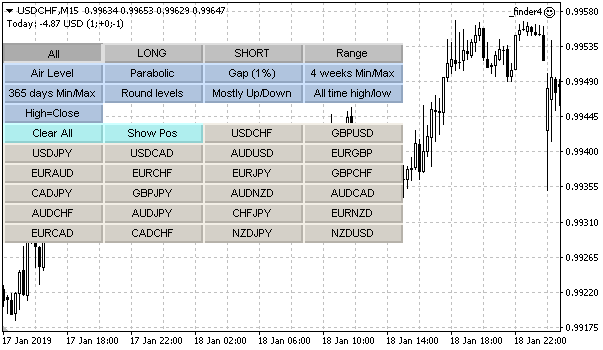```void show_symbols(){

// 初始化用于定义 X 和 Y 坐标的变量
int btn_left=0;
int btn_right=(int) ChartGetInteger(0, CHART_WIDTH_IN_PIXELS)-77;
btn_line++;

if( cur_panel==0 ){
ObjectCreate(0, exprefix+"clear_"+(string) cur_panel, OBJ_BUTTON, 0, 0, 0);
ObjectSetInteger(0,exprefix+"clear_"+(string) cur_panel,OBJPROP_XDISTANCE,btn_left);
ObjectSetInteger(0,exprefix+"clear_"+(string) cur_panel,OBJPROP_YDISTANCE,BTN_HEIGHT*btn_line);
ObjectSetInteger(0,exprefix+"clear_"+(string) cur_panel,OBJPROP_XSIZE,BTN_WIDTH);
ObjectSetInteger(0,exprefix+"clear_"+(string) cur_panel,OBJPROP_YSIZE,BTN_HEIGHT);
ObjectSetInteger(0,exprefix+"clear_"+(string) cur_panel,OBJPROP_FONTSIZE,8);
ObjectSetInteger(0,exprefix+"clear_"+(string) cur_panel,OBJPROP_COLOR,clrBlack);
ObjectSetInteger(0,exprefix+"clear_"+(string) cur_panel,OBJPROP_BGCOLOR,clrPaleTurquoise);
ObjectSetInteger(0,exprefix+"clear_"+(string) cur_panel,OBJPROP_SELECTABLE,false);
ObjectSetString(0,exprefix+"clear_"+(string) cur_panel,OBJPROP_TEXT,"Clear All");
btn_left+=BTN_WIDTH;
ObjectCreate(0, exprefix+"showpos", OBJ_BUTTON, 0, 0, 0);
ObjectSetInteger(0,exprefix+"showpos",OBJPROP_XDISTANCE,btn_left);
ObjectSetInteger(0,exprefix+"showpos",OBJPROP_YDISTANCE,BTN_HEIGHT*btn_line);
ObjectSetInteger(0,exprefix+"showpos",OBJPROP_XSIZE,BTN_WIDTH);
ObjectSetInteger(0,exprefix+"showpos",OBJPROP_YSIZE,BTN_HEIGHT);
ObjectSetInteger(0,exprefix+"showpos",OBJPROP_FONTSIZE,8);
ObjectSetInteger(0,exprefix+"showpos",OBJPROP_COLOR,clrBlack);
ObjectSetInteger(0,exprefix+"showpos",OBJPROP_BGCOLOR,clrPaleTurquoise);
ObjectSetInteger(0,exprefix+"showpos",OBJPROP_SELECTABLE,false);
ObjectSetString(0,exprefix+"showpos",OBJPROP_TEXT,"Show Pos");
btn_left+=BTN_WIDTH;
}else if( cur_panel<ArraySize(arrPanels) && arrPanels[cur_panel].Total()>0 ){
ObjectCreate(0, exprefix+"clear_"+(string) cur_panel, OBJ_BUTTON, 0, 0, 0);
ObjectSetInteger(0,exprefix+"clear_"+(string) cur_panel,OBJPROP_XDISTANCE,btn_left);
ObjectSetInteger(0,exprefix+"clear_"+(string) cur_panel,OBJPROP_YDISTANCE,BTN_HEIGHT*btn_line);
ObjectSetInteger(0,exprefix+"clear_"+(string) cur_panel,OBJPROP_XSIZE,BTN_WIDTH);
ObjectSetInteger(0,exprefix+"clear_"+(string) cur_panel,OBJPROP_YSIZE,BTN_HEIGHT);
ObjectSetInteger(0,exprefix+"clear_"+(string) cur_panel,OBJPROP_FONTSIZE,8);
ObjectSetInteger(0,exprefix+"clear_"+(string) cur_panel,OBJPROP_COLOR,clrBlack);
ObjectSetInteger(0,exprefix+"clear_"+(string) cur_panel,OBJPROP_BGCOLOR,clrPaleTurquoise);
ObjectSetInteger(0,exprefix+"clear_"+(string) cur_panel,OBJPROP_SELECTABLE,false);
ObjectSetString(0,exprefix+"clear_"+(string) cur_panel,OBJPROP_TEXT,"Clear");
btn_left+=BTN_WIDTH;
ObjectCreate(0, exprefix+"new_"+(string) cur_panel, OBJ_BUTTON, 0, 0, 0);
ObjectSetInteger(0,exprefix+"new_"+(string) cur_panel,OBJPROP_XDISTANCE,btn_left);
ObjectSetInteger(0,exprefix+"new_"+(string) cur_panel,OBJPROP_YDISTANCE,BTN_HEIGHT*btn_line);
ObjectSetInteger(0,exprefix+"new_"+(string) cur_panel,OBJPROP_XSIZE,BTN_WIDTH);
ObjectSetInteger(0,exprefix+"new_"+(string) cur_panel,OBJPROP_YSIZE,BTN_HEIGHT);
ObjectSetInteger(0,exprefix+"new_"+(string) cur_panel,OBJPROP_FONTSIZE,8);
ObjectSetInteger(0,exprefix+"new_"+(string) cur_panel,OBJPROP_COLOR,clrBlack);
ObjectSetInteger(0,exprefix+"new_"+(string) cur_panel,OBJPROP_BGCOLOR,clrPaleTurquoise);
ObjectSetInteger(0,exprefix+"new_"+(string) cur_panel,OBJPROP_SELECTABLE,false);
ObjectSetString(0,exprefix+"new_"+(string) cur_panel,OBJPROP_TEXT,"Open All");
btn_left+=BTN_WIDTH;
}

// 如果当前打开的选项卡索引超过了 homework 选项卡数组元素的元素数
// 加上所有交易品种的选项卡
// 则这就是自动挑选选项卡
if(cur_panel>(ArraySize(arrPanels)-1)){
MqlRates rates[];
ArraySetAsSeries(rates, true);
arrTT.Resize(0);

CArrayString tmpSymbols;
if( tmpNumAddon==0 && air_only_home ){
for( int j=1; j<ArraySize(arrPanels); j++ ){
for( int k=0; k<arrPanels[j].Total(); k++ ){
string curName=arrPanels[j].At(k);
bool isYes=false;
for( int r=0; r<tmpSymbols.Total(); r++ ){
if(tmpSymbols.At(r)==curName){
isYes=true;
break;
}
}
if(!isYes){
}
}
}
}else{
if( ArraySize(result)>1 ){
for(int j=0;j<ArraySize(result);j++){
StringReplace(result[j], " ", "");
if(StringLen(result[j])<1){
continue;
}
}
}else{
for( int i=0; i<SymbolsTotal(addons_infowatch); i++ ){
}
}
}

case 0: // 水平
// 选项卡内容显示代码
break;
case 1: // 抛物线
// 选项卡内容显示代码
break;
case 2: // 跳空
// 选项卡内容显示代码
break;
case 3: //4周最小值/最大值
// 选项卡内容显示代码
break;
case 4: //365 天最小值/最大值
// 选项卡内容显示代码
break;
case 5: // 近似水平
// 选项卡内容显示代码
break;
case 6: // 最上升/下降
// 选项卡内容显示代码
break;
case 7: // 所有时间最高价/最低价
// 选项卡内容显示代码
break;
case 8: // 最高价=收盘价
// 选项卡内容显示代码
break;
}

// 数组中的每个交易品种都在图表中显示一个按钮
// 按钮上加上交易品种名称
for( int i=0; i<addonArr.Total(); i++ ){

if( btn_left>btn_right-BTN_WIDTH ){
btn_line++;
btn_left=0;
}

ObjectCreate(0, exprefix+"btn"+(string) i, OBJ_BUTTON, 0, 0, 0);
ObjectSetInteger(0,exprefix+"btn"+(string) i,OBJPROP_XDISTANCE,btn_left);
ObjectSetInteger(0,exprefix+"btn"+(string) i,OBJPROP_YDISTANCE,BTN_HEIGHT*btn_line);
ObjectSetInteger(0,exprefix+"btn"+(string) i,OBJPROP_XSIZE,BTN_WIDTH);
ObjectSetInteger(0,exprefix+"btn"+(string) i,OBJPROP_YSIZE,BTN_HEIGHT);
ObjectSetInteger(0,exprefix+"btn"+(string) i,OBJPROP_FONTSIZE,8);
ObjectSetInteger(0,exprefix+"btn"+(string) i,OBJPROP_COLOR,clrBlack);
ObjectSetInteger(0,exprefix+"btn"+(string) i,OBJPROP_SELECTABLE,false);
if( arrTT.At(i)>0 ){
ObjectSetString(0,exprefix+"btn"+(string) i,OBJPROP_TOOLTIP,(string) arrTT.At(i));
}

ObjectSetInteger(0,exprefix+"btn"+(string) i,OBJPROP_BGCOLOR,clrPeachPuff);
}

btn_left+=BTN_WIDTH;
}

// 因为按钮已经显示，退出函数
return;
}

// 在图表上显示数组中每个交易品种的按钮
// 当前活动选项卡
// 按钮上加上交易品种名称
for( int i=0; i<arrPanels[cur_panel].Total(); i++ ){

if( btn_left>btn_right-BTN_WIDTH ){
btn_line++;
btn_left=0;
}

ObjectCreate(0, exprefix+"btn"+(string) i, OBJ_BUTTON, 0, 0, 0);
ObjectSetInteger(0,exprefix+"btn"+(string) i,OBJPROP_XDISTANCE,btn_left);
ObjectSetInteger(0,exprefix+"btn"+(string) i,OBJPROP_YDISTANCE,BTN_HEIGHT*btn_line);
ObjectSetInteger(0,exprefix+"btn"+(string) i,OBJPROP_XSIZE,BTN_WIDTH);
ObjectSetInteger(0,exprefix+"btn"+(string) i,OBJPROP_YSIZE,BTN_HEIGHT);
ObjectSetInteger(0,exprefix+"btn"+(string) i,OBJPROP_FONTSIZE,8);
ObjectSetInteger(0,exprefix+"btn"+(string) i,OBJPROP_COLOR,clrBlack);
ObjectSetString(0,exprefix+"btn"+(string) i,OBJPROP_TEXT,arrPanels[cur_panel].At(i));
ObjectSetInteger(0,exprefix+"btn"+(string) i,OBJPROP_SELECTABLE,false);

if( !noSYMBwithPOS || cur_panel>0 ){
if( checkSYMBwithPOS(arrPanels[cur_panel].At(i)) ){
ObjectSetInteger(0,exprefix+"btn"+(string) i,OBJPROP_BGCOLOR,clrPeachPuff);
}
}

btn_left+=BTN_WIDTH;
}

}
```

```            for( int i=0; i<tmpSymbols.Total(); i++ ){
// 如果交易品种应当显示，定义的代码
}
```

### 空中水平 (盘整范围)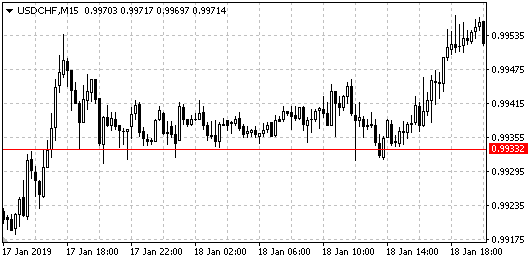```sinput string        delimeter_06=""; // --- 额外的空中水平选项卡 ---
input bool           air_level_m5=true; // 在 M5 上寻找空中水平
input bool           air_level_m15=true; // 在 M15 上寻找空中水平
input bool           air_level_m30=false; // 在 M30 上寻找空中水平
input bool           air_level_h1=false; // 在 H1 上寻找空中水平
input bool           air_level_h4=false; // 在 H4 上寻找空中水平
input bool           air_level_d1=false; // 在 D1 上寻找空中水平
```

```input uchar          air_level_count=4; // Number of level bars（水平的柱数）
input uchar          air_level_offset=3; // Offset relative to level in points（相对水平的点数偏移）
input bool           air_level_cur_day=true; // Only levels in the current day's direction（只有当天方向的水平）
input bool           air_level_prev_day=true; // Only levels in the previous day's direction（只有前一天方向上的水平）
input bool           air_only_home=false; // Search only in "homework"（只在 "homework" 中搜索）
```

Number of level bars 参数让我们指定触及最近价格的柱数以假定这是个平盘的范围，这个参数的最佳值是4个柱。柱数越大，平盘范围的质量就越高，但是找到它们的频率就越低。

Offset relative to level in points 参数让我们指定范围的点数，也就是价格被认为“位置很近”，换句话说，如果参数为0，柱应当正好触及相同的价格，一般来说，这种情况很少，特别是在较高的时间框架上。在大多数情况下，价格无法达到指定的水平，因此，默认参数值为3点。

```               if(air_level_m5 && CopyRates(addonName, PERIOD_M5, 0, air_level_count+1, rates)==air_level_count+1){
if( (!air_level_cur_day || getmeinfoday_symbol(addonName, 0)<=0) && (!air_level_prev_day || getmeinfoday_symbol(addonName, 1)<=0) && rates.high<rates.high ){
bool isOk=true;
for( int j=1; j<air_level_count; j++ ){
if( MathAbs(rates.high-rates[j+1].high) <= air_level_offset*SymbolInfoDouble(addonName, SYMBOL_POINT) ){
}else{
isOk=false;
}
}
}
}else if( (!air_level_cur_day || getmeinfoday_symbol(addonName, 0)>=0) && (!air_level_prev_day || getmeinfoday_symbol(addonName, 1)>=0) && rates.low>rates.low ){
bool isOk=true;
for( int j=1; j<air_level_count; j++ ){
if( MathAbs(rates.low-rates[j+1].low) <= air_level_offset*SymbolInfoDouble(addonName, SYMBOL_POINT) ){
}else{
isOk=false;
}
}
}
}
}
```

### 抛物线弧形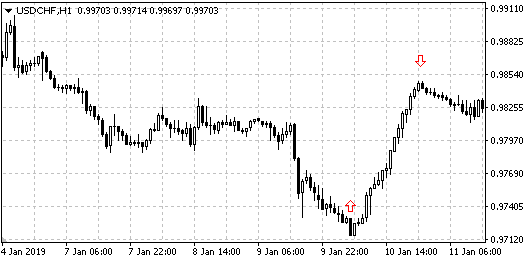```               if(CopyRates(addonName, PERIOD_M5, 0, 6, rates)==6){
if( rates.low>rates.low && rates.low>rates.low && rates.low<=rates.low && rates.low<=rates.low && rates.low<=rates.low ){
}
}else if( rates.high<rates.high && rates.high<rates.high && rates.high>=rates.high && rates.high>=rates.high && rates.high>=rates.high ){
}
}
}
```

### 缺口

```               if(CopyRates(addonName, PERIOD_D1, 0, 2, rates)==2){
if( rates.open>rates.close+(rates.open*(gap_min/100)) || rates.open<rates.close-(rates.open*(gap_min/100)) ){
}
}
}
```

### 4周最高/最低

4 周最高/最低 选项卡所提供的交易品种列表是，它们的当前价格在四周内的最高点/最低点。它的代码如下:

```               if(CopyRates(addonName, PERIOD_W1, 0, 4, rates)==4){
bool newMin=true;
bool newMax=true;
if( rates.close!=rates.high && rates.close!=rates.low ){
newMin=false;
newMax=false;
}else{
for( int j=1; j<4; j++ ){
if( rates.high < rates[j].high ){
newMax=false;
}
if( rates.low > rates[j].low ){
newMin=false;
}
}
}
if( newMin || newMax ){
}
}
}
```

### 年度最高/最低

```               if(CopyRates(addonName, PERIOD_W1, 0, 52, rates)==52){
bool newMin=true;
bool newMax=true;
if( rates.close!=rates.high && rates.close!=rates.low ){
newMin=false;
newMax=false;
}else{
for( int j=1; j<52; j++ ){
if( rates.high < rates[j].high ){
newMax=false;
}
if( rates.low > rates[j].low ){
newMin=false;
}
}
}
if( newMin || newMax ){
}
}
}
```

### 价格接近取整水平

```               switch((int) SymbolInfoInteger(addonName, SYMBOL_DIGITS)){
case 0:
break;
case 2:
if(CopyRates(addonName, PERIOD_M5, 0, 1, rates)==1){
double tmpRound=rates.close - (int) rates.close;
if( (tmpRound>0.46 && tmpRound<0.54) || tmpRound>0.96 || tmpRound<0.04 ){
}
}
}
break;
}
```

### 多数时间上涨/下跌

```sinput string        delimeter_08=""; // --- 额外的多数上涨/下跌选项卡 ---
input int            mostly_count=15; // Check the last specified number of days（检查最近指定的天数）
input int            mostly_percent=90; // Specified percentage in one direction exceeded（指定在一个方向上超过的百分比）
```

Check the last specified number of days 参数可以定义寻找所需资产工具的天数，换句话说，我们大多数情况会在D1中寻找一个方向上的变化。

Specified percentage in one direction exceeded 参数可以指定最小百分比，就是相互超过的百分比。默认值是 90，这表示，为了达到这个选项卡，在指定期间内的价格变化应该在90%以上的天数在某个方向上变化。

```               if(CopyRates(addonName, PERIOD_D1, 1, mostly_count, rates)==mostly_count){
int mostlyLong=0;
int mostlyShort=0;
for( int j=0; j<mostly_count; j++ ){
if(rates[j].close>rates[j].open){
mostlyLong++;
}else if(rates[j].close<rates[j].open){
mostlyShort++;
}
}
if( !mostlyLong || !mostlyShort ){
}else if( ((mostlyLong*100)/(mostlyLong+mostlyShort)) >= mostly_percent ){
}else if( ((mostlyShort*100)/(mostlyLong+mostlyShort)) >= mostly_percent ){
}
}
```

### 每个柱有新高/低

```sinput string        delimeter_09=""; // --- 额外的 总有新高/低选项卡 ---
input ENUM_TIMEFRAMES alltime_period=PERIOD_D1; // 时段
input int            alltime_count=15; // Check the last specified number of bars（检查最近指定的柱数）
```

```               if(CopyRates(addonName, alltime_period, 1, alltime_count, rates)==alltime_count){
bool alltimeHigh=true;
bool alltimeLow=true;
for( int j=1; j<alltime_count; j++ ){
if(rates[j].high>rates[j-1].high){
alltimeHigh=false;
}
if(rates[j].low<rates[j-1].low){
alltimeLow=false;
}
}
if( alltimeHigh || alltimeLow ){
}
}
```

### 交易日在当日高点/低点结束

• before a session starts – 前一天收盘时处于高点/低点的交易品种；
• after a session starts – 当前处于当日高点/低点的交易品种。

```sinput string        delimeter_10=""; // --- 额外的 最高价=收盘价 选项卡 ---
input ENUM_TIMEFRAMES highclose_period=PERIOD_D1; // 时段
input int            highclose_offset=0; // 最高价/最低价偏移点数
```

```               if(CopyRates(addonName, highclose_period, 0, 1, rates)==1){
if( rates.close+highclose_offset >= rates.high ){
}else if( rates.close-highclose_offset <= rates.low ){
}
}
```

### 添加自定义选项卡

```         case index: // 选项卡名称
for( int i=0; i<tmpSymbols.Total(); i++ ){

// 检查代码
}

break;
```

### 结论

finder.ex5 (270.41 KB)
finder.mq5 (186.66 KB)
finder4.ex4 (178.96 KB)
finder4.mq4 (186.66 KB)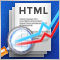利用 HTML 报告分析交易结果

MetaTrader 5 平台具有保存交易报告，以及智能交易系统测试和优化报告的功能。 交易和测试报告可以按照两种格式保存：XLSX 和 HTML，而优化报告可以保存为 XML。 在本文中，我们将研究 HTML 测试报告，XML 优化报告和 HTML 交易历史报告。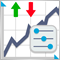分离策略在趋势和盘整条件下的优化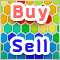在算法交易中 KOHONEN 神经网络的实际应用 第二部分优化和预测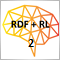蒙特卡罗方法在强化学习中的应用